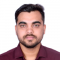## How to unset 'sharex' or 'sharey' from two axes in Matplotlib?Rishikesh Kumar Rishi

Updated on 02-Feb-2022 11:13:03

To inset sharex and sharey from two axes in matplotlib, we can use 'none', i.e., False or 'none'. Each subplot X- or Y-axis will be independent.StepsSet the figure size and adjust the padding between and around the subplots.Initialize two variables rows and cols.Create a figure and a set of subplots.Iterate ... Read More

## How to obtain 3D colored surface via Python?Rishikesh Kumar Rishi

Updated on 02-Feb-2022 10:55:15

To obtain 3D colored surface via Python, we can take the following steps −StepsSet the figure size and adjust the padding between and around the subplots.Create x and y data points using numpy.Get 3D data, i.e., z.Create a new figure or activate an existing figure.Get the 3D axes.Create a surface ... Read More

## How to set the xticklabels for date in matplotlib?Rishikesh Kumar Rishi

Updated on 02-Feb-2022 10:51:20

To set the xticklabels for date in matplotlib, we can take the following steps −StepsSet the figure size and adjust the padding between and around the subplots.Create two lists of epochs and values.Get a list of dates from epochs.Create a figure and a set of subplots.Plot the date and values ... Read More

## Scatter a 2D numpy array in matplotlibRishikesh Kumar Rishi

Updated on 02-Feb-2022 10:47:22

To scatter a 2D numpy array in matplotlib, we can take the following steps −StepsSet the figure size and adjust the padding between and around the subplots.Create random data of 100×3 dimension.Use the scatter() method to plot 2D numpy array, i.e., data.To display the figure, use show() method.Exampleimport numpy as ... Read More

## How to avoid overlapping error bars in matplotlib?Rishikesh Kumar Rishi

Updated on 02-Feb-2022 10:44:17

To avoid overlapping error bars in matplotlib, we can take the following steps −StepsSet the figure size and adjust the padding between and around the subplots.Create a list of names.Get the data points for y1 and y2, and errors ye1, ye2.Create a figure and a set of subplots.Create a mutable ... Read More

## How do I remove the Y-axis from a Pylab-generated picture?Rishikesh Kumar Rishi

Updated on 02-Feb-2022 10:40:17

To remove the Y-axis from a Pylab-generated picture, we can get the current axis of the plot and use the set_visible(False) method.StepsSet the figure size and adjust the padding between and around the subplots.Create x and y data points using numpy.Plot the x and y data points using plot() method.Get ... Read More

## Flushing all current figures in matplotlibRishikesh Kumar Rishi

Updated on 02-Feb-2022 10:33:33

To flush all current figures in matplotlib, use close('all') method.StepsSet the figure size and adjust the padding between and around the subplots.Create a figure with the title "First Figure".Create another figure with the title "Second Figure".To close all figures, use close('all').To display the figure, use show() method.Examplefrom matplotlib import pyplot ... Read More

## How to create multiple series scatter plots with connected points using seaborn?Rishikesh Kumar Rishi

Updated on 02-Feb-2022 10:28:25

To create multiple series scatter plots with connected points using seaborn, we can take the following steps −StepsSet the figure size and adjust the padding between and around the subplots.Create a Pandas data frame of two-dimensional, size-mutable, potentially heterogeneous tabular data.Multi-plot grid for plotting conditional relationships.Apply a plotting function to ... Read More

## How to make a histogram with bins of equal area in Matplotlib?Rishikesh Kumar Rishi

Updated on 02-Feb-2022 10:13:04

To make a histogram with bins of equal area in matplotlib, we can take the following steps −StepsSet the figure size and adjust the padding between and around the subplots.Create random data points using numpy.Plot a histogram with equal_area method that makes an equal area of the patches.To display the ... Read More

## Combining two heatmaps in seabornRishikesh Kumar Rishi

Updated on 02-Feb-2022 10:08:49

To combine two heatmaps in seaborn, we can take the following steps −StepsSet the figure size and adjust the padding between and around the subplots.Create two Pandas data frames.Create a figure and a set of subplots, ax1 and ax2.Plot the rectangular data as a color-encoded matrix, on ax1 and ax2.Move ... Read More